The Central Limit Theorem

# 36 The Central Limit Theorem for Proportions

The Central Limit Theorem tells us that the point estimate for the sample mean,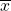, comes from a normal distribution of‘s. This theoretical distribution is called the sampling distribution of‘s. We now investigate the sampling distribution for another important parameter we wish to estimate; p from the binomial probability density function.

If the random variable is discrete, such as for categorical data, then the parameter we wish to estimate is the population proportion. This is, of course, the probability of drawing a success in any one random draw. Unlike the case just discussed for a continuous random variable where we did not know the population distribution of X’s, here we actually know the underlying probability density function for these data; it is the binomial. The random variable is X = the number of successes and the parameter we wish to know is p, the probability of drawing a success which is of course the proportion of successes in the population. The question at issue is: from what distribution was the sample proportion,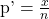drawn? The sample size is n and X is the number of successes found in that sample. This is a parallel question that was just answered by the Central Limit Theorem: from what distribution was the sample mean,, drawn? We saw that once we knew that the distribution was the Normal distribution then we were able to create confidence intervals for the population parameter, µ. We will also use this same information to test hypotheses about the population mean later. We wish now to be able to develop confidence intervals for the population parameter “p” from the binomial probability density function.

In order to find the distribution from which sample proportions come we need to develop the sampling distribution of sample proportions just as we did for sample means. So again imagine that we randomly sample say 50 people and ask them if they support the new school bond issue. From this we find a sample proportion, p’, and graph it on the axis of p’s. We do this again and again etc., etc. until we have the theoretical distribution of p’s. Some sample proportions will show high favorability toward the bond issue and others will show low favorability because random sampling will reflect the variation of views within the population. What we have done can be seen in (Figure). The top panel is the population distributions of probabilities for each possible value of the random variable X. While we do not know what the specific distribution looks like because we do not know p, the population parameter, we do know that it must look something like this. In reality, we do not know either the mean or the standard deviation of this population distribution, the same difficulty we faced when analyzing the X’s previously.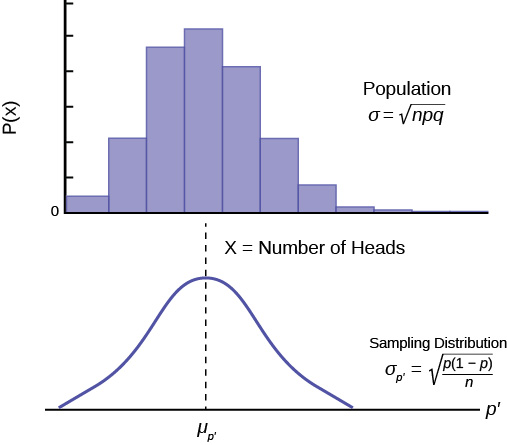(Figure) places the mean on the distribution of population probabilities as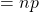but of course we do not actually know the population mean because we do not know the population probability of success,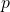. Below the distribution of the population values is the sampling distribution of‘s. Again the Central Limit Theorem tells us that this distribution is normally distributed just like the case of the sampling distribution for‘s. This sampling distribution also has a mean, the mean of the‘s, and a standard deviation,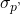.

Importantly, in the case of the analysis of the distribution of sample means, the Central Limit Theorem told us the expected value of the mean of the sample means in the sampling distribution, and the standard deviation of the sampling distribution. Again the Central Limit Theorem provides this information for the sampling distribution for proportions. The answers are:

1. The expected value of the mean of sampling distribution of sample proportions,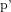, is the population proportion, p.
2. The standard deviation of the sampling distribution of sample proportions,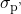, is the population standard deviation divided by the square root of the sample size, n.

Both these conclusions are the same as we found for the sampling distribution for sample means. However in this case, because the mean and standard deviation of the binomial distribution both rely upon, the formula for the standard deviation of the sampling distribution requires algebraic manipulation to be useful. We will take that up in the next chapter. The proof of these important conclusions from the Central Limit Theorem is provided below.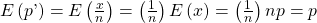(The expected value of X, E(x), is simply the mean of the binomial distribution which we know to be np.)The standard deviation of the sampling distribution for proportions is thus: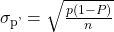Parameter Population distribution Sample Sampling distribution of p’s
Mean µ = np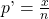p’ and E(p’) = p
Standard Deviation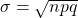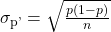(Figure) summarizes these results and shows the relationship between the population, sample and sampling distribution. Notice the parallel between this Table and Table 7.1 for the case where the random variable is continuous and we were developing the sampling distribution for means.

Reviewing the formula for the standard deviation of the sampling distribution for proportions we see that as n increases the standard deviation decreases. This is the same observation we made for the standard deviation for the sampling distribution for means. Again, as the sample size increases, the point estimate for either µ or p is found to come from a distribution with a narrower and narrower distribution. We concluded that with a given level of probability, the range from which the point estimate comes is smaller as the sample size, n, increases. Figure 7.8 shows this result for the case of sample means. Simply substitute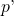forand we can see the impact of the sample size on the estimate of the sample proportion.

### Chapter Review

The Central Limit Theorem can also be used to illustrate that the sampling distribution of sample proportions is normally distributed with the expected value of p and a standard deviation ofA question is asked of a class of 200 freshmen, and 23% of the students know the correct answer. If a sample of 50 students is taken repeatedly, what is the expected value of the mean of the sampling distribution of sample proportions?

0.23

A question is asked of a class of 200 freshmen, and 23% of the students know the correct answer. If a sample of 50 students is taken repeatedly, what is the standard deviation of the mean of the sampling distribution of sample proportions?

0.060

A game is played repeatedly. A player wins one-fifth of the time. If samples of 40 times the game is played are taken repeatedly, what is the expected value of the mean of the sampling distribution of sample proportions?

1/5

A game is played repeatedly. A player wins one-fifth of the time. If samples of 40 times the game is played are taken repeatedly, what is the standard deviation of the mean of the sampling distribution of sample proportions?

0.063

A virus attacks one in three of the people exposed to it. An entire large city is exposed. If samples of 70 people are taken, what is the expected value of the mean of the sampling distribution of sample proportions?

1/3

A virus attacks one in three of the people exposed to it. An entire large city is exposed. If samples of 70 people are taken, what is the standard deviation of the mean of the sampling distribution of sample proportions?

0.056

A company inspects products coming through its production process, and rejects detected products. One-tenth of the items are rejected. If samples of 50 items are taken, what is the expected value of the mean of the sampling distribution of sample proportions?

1/10

A company inspects products coming through its production process, and rejects detected products. One-tenth of the items are rejected. If samples of 50 items are taken, what is the standard deviation of the mean of the sampling distribution of sample proportions?

0.042

### Homework

A farmer picks pumpkins from a large field. The farmer makes samples of 260 pumpkins and inspects them. If one in fifty pumpkins are not fit to market and will be saved for seeds, what is the standard deviation of the mean of the sampling distribution of sample proportions?

0.0087

A store surveys customers to see if they are satisfied with the service they received. Samples of 25 surveys are taken. One in five people are unsatisfied. What is the variance of the mean of the sampling distribution of sample proportions for the number of unsatisfied customers? What is the variance for satisfied customers?

0.0064, 0.0064

A company gives an anonymous survey to its employees to see what percent of its employees are happy. The company is too large to check each response, so samples of 50 are taken, and the tendency is that three-fourths of the employees are happy. For the mean of the sampling distribution of sample proportions, answer the following questions, if the sample size is doubled.

1. How does this affect the mean?
2. How does this affect the standard deviation?
3. How does this affect the variance?
1. It has no effect.
2. It is divided by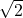.
3. It is divided by 2.

A pollster asks a single question with only yes and no as answer possibilities. The poll is conducted nationwide, so samples of 100 responses are taken. There are four yes answers for each no answer overall. For the mean of the sampling distribution of sample proportions, find the following for yes answers.

1. The expected value.
2. The standard deviation.
3. The variance.
1. 4/5
2. 0.04
3. 0.0016

The mean of the sampling distribution of sample proportions has a value of p of 0.3, and sample size of 40.

1. Is there a difference in the expected value if p and q reverse roles?
2. Is there a difference in the calculation of the standard deviation with the same reversal?
1. Yes
2. No